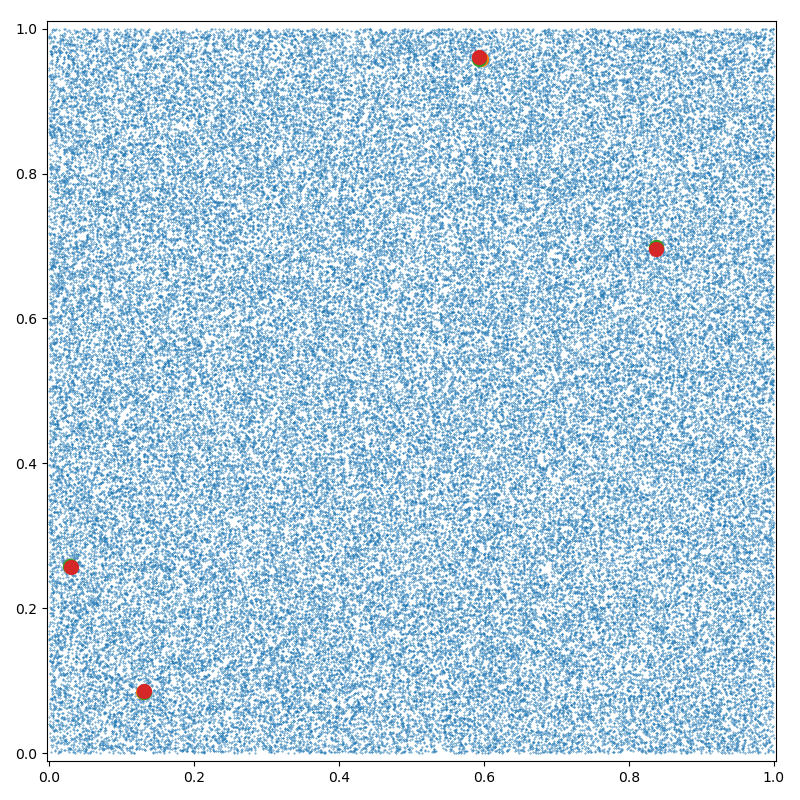# Arg-K-Min reduction

Using the pykeops.numpy API, we define a dataset of N points in $$\mathbb R^D$$ and compute for each point the indices of its K nearest neighbours (including itself).

## Setup

Standard imports:

import time

import matplotlib.pyplot as plt
import numpy as np

from pykeops.numpy import Genred


Define our dataset:

N = 100000  # Number of points
D = 2  # Dimension of the ambient space
K = 3  # Number of neighbors to look for

dtype = "float32"  # May be 'float32' or 'float64'

x = np.random.rand(N, D).astype(dtype)


## KeOps Kernel

formula = "SqDist(x,y)"  # Use a simple Euclidean (squared) norm
variables = [
"x = Vi(" + str(D) + ")",  # First arg : i-variable, of size D
"y = Vj(" + str(D) + ")",
]  # Second arg: j-variable, of size D

# N.B.: The number K is specified as an optional argument opt_arg
my_routine = Genred(formula, variables, reduction_op="ArgKMin", axis=1, opt_arg=K)


Using our new pykeops.numpy.Genred routine, we perform a K-nearest neighbor search ( reduction_op = "ArgKMin" ) over the $$j$$ variable $$y_j$$ ( axis = 1):

Note

If CUDA is available and backend is "auto" or not specified, KeOps will:

1. Load the data on the GPU

2. Perform the computation on the device

3. Unload the result back to the CPU

as it is assumed to be most efficient for large-scale problems. By specifying backend = "CPU" in the call to my_routine, you can bypass this procedure and use a simple C++ for loop instead.

# Dummy first call to warm-up the GPU and thus get an accurate timing:
my_routine(np.random.rand(10, D).astype(dtype), np.random.rand(10, D).astype(dtype))

# Actually perform our K-nn search:
start = time.time()
ind = my_routine(x, x, backend="auto")
print("Time to perform the K-nn search: ", round(time.time() - start, 5), "s")

# The result is now an (N,K) array of integers:
print("Output values :")
print(ind)

plt.figure(figsize=(8, 8))
plt.scatter(x[:, 0], x[:, 1], s=25 * 500 / len(x))

for k in range(K):  # Highlight some points and their nearest neighbors
plt.scatter(x[ind[:4, k], 0], x[ind[:4, k], 1], s=100)

plt.axis("equal")
plt.axis([0, 1, 0, 1])
plt.tight_layout()
plt.show()Time to perform the K-nn search:  0.01061 s
Output values :
[[    0 20639 57446]
[    1 12439 37977]
[    2 52564 30564]
...
[99997 30406 77233]
[99998 37431 22574]
[99999 16563 64568]]


Total running time of the script: ( 0 minutes 0.223 seconds)

Gallery generated by Sphinx-Gallery